Project tutorial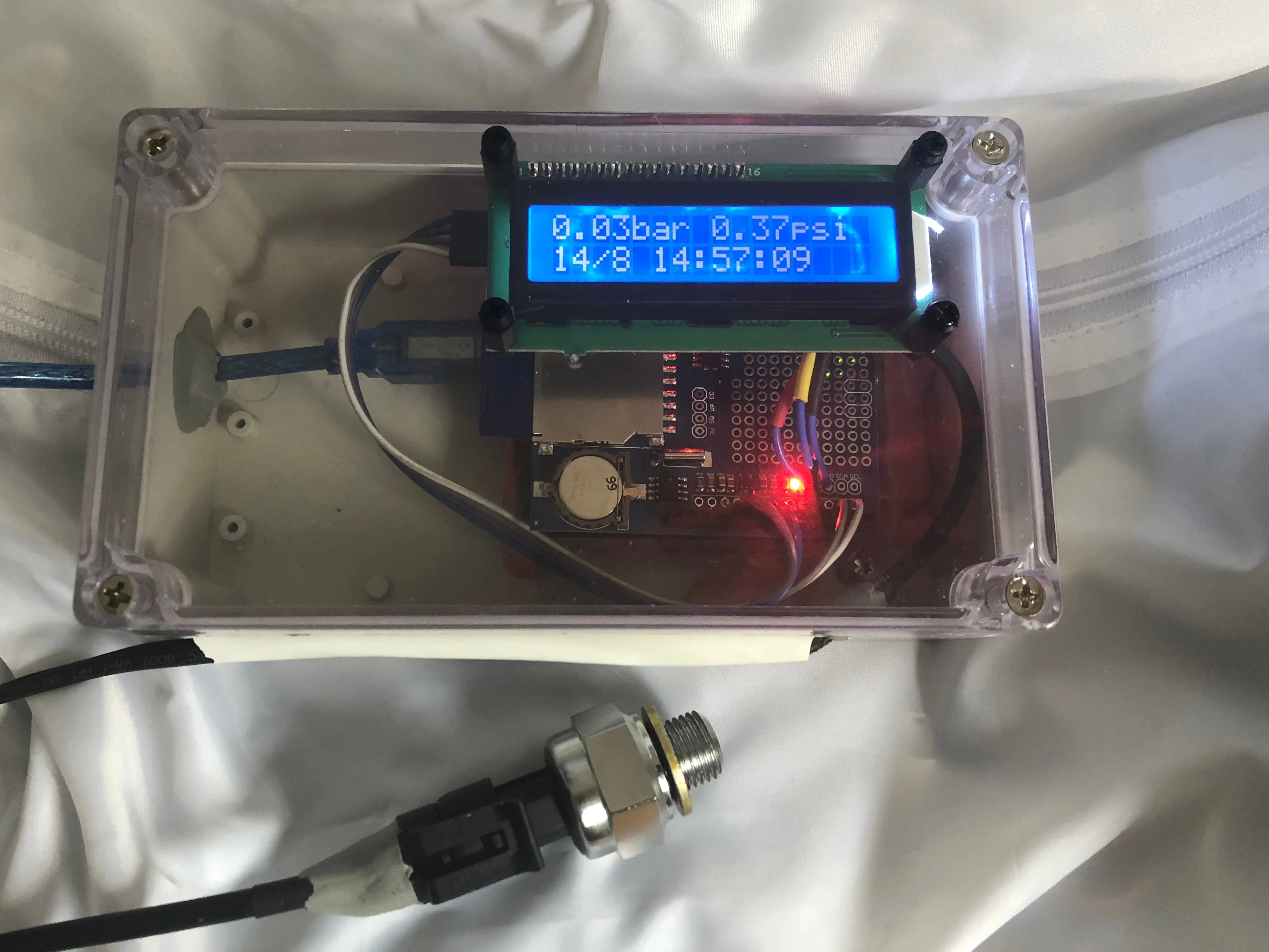# Arduino Pressure Measuring and Logging © GPL3+

An SKU237545 pressure sensor.

• 26,146 views
• 20 respects

## Components and suppliesArduino UNO & Genuino UNO
×1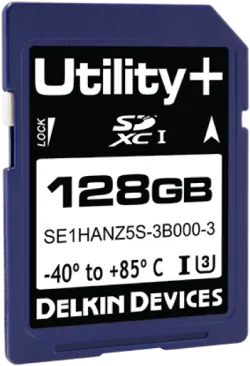Delkin Commercial MLC SD
×1
 pressure sensor SKU237545
×1
 data logger rtc
×1
 i2c lcd
×1
 Waterproof Clear Cover Plastic Electronic Project Box 158 x 90 x 60mm
×1

## Apps and online services

This project was the result of wanting to record air pressure as built in a reservoir to check the efficiency of a mechanical compressor.

Help from George Kontaxis

## Code

##### SKU237545 Pressure Sensor with lcd realtime clock and data loggingC/C++
```//created 12th August 2018 Philip Blayney & Jack Davidson using code from George //Kontaxis for the SKU237545 Pressure Sensor

#include "LiquidCrystal_PCF8574.h"
#include "RTClib.h"         // real time clock
#include <SD.h>            // sd card

#define LCD_ADDRESS 0x27                                                                              // Define LCD characteristics
#define LCD_ROWS 2
#define LCD_COLUMNS 16
#define SCROLL_DELAY 150
#define BACKLIGHT 255                                                                                // object initialization
LiquidCrystal_PCF8574 lcdI2C;
RTC_DS1307 RTC; // define the Real Time Clock object
int n = 1;
int val;                                                                                             // A simple data logger for the Arduino analog pins
#define LOG_INTERVAL  1000 // mills between entries (reduce to take more/faster data)
// how many milliseconds before writing the logged data permanently to disk
// set it to the LOG_INTERVAL to write each time (safest)
// set it to 10*LOG_INTERVAL to write all data every 10 datareads, you could lose up to
// the last 10 reads if power is lost but it uses less power and is much faster!

#define SYNC_INTERVAL 10000 // mills between calls to flush() - to write data to the card 600000 = 10 minutes
uint32_t syncTime = 0; // time of last sync()

const int chipSelect = 10;                                                                           // for the data logging shield, we use digital pin 10 for the SD cs line

File logfile;

void error(char *str)
{
Serial.print(F("error: "));
Serial.println(str);
}

void setup(void)
{

Serial.begin(9600);                                                                                 // start serial port
Serial.println(F("pressure reading off SKU237545 SENSOR"));                                         //F = macro function to save dynamic memory
lcdI2C.print(F("SKU237545 SENSOR" ));
lcdI2C.setCursor(0,1);
lcdI2C.print(F("  bar and psi " ));

delay (2000);

// initialize the SD card
Serial.print(F("Initializing SD card..."));
// make sure that the default chip select pin is set to
// output, even if you don't use it:
pinMode(10, OUTPUT);

if (!SD.begin(chipSelect)) {                                                                     // see if the card is present and can be initialized:
error("Card failed, or not present");
}
Serial.println(F("card initialized."));

char filename[] = "LOGGER00.CSV";                                                                // create a new file
for (uint8_t i = 0; i < 100; i++) {
filename = i/10 + '0';
filename = i%10 + '0';
if (! SD.exists(filename)) {
// only open a new file if it doesn't exist
logfile = SD.open(filename, FILE_WRITE);
break;  // leave the loop!
}
}

if (! logfile) {
error("couldnt create file");
}
lcdI2C.clear();
lcdI2C.setCursor(0,0);
lcdI2C.print(F("File No " ));
lcdI2C.print(filename);
Serial.print(F("Logging to: "));
Serial.println(filename);

// connect to RTC

if (!RTC.begin()) {
logfile.println("RTC failed");
#if ECHO_TO_SERIAL
Serial.println(F("RTC failed"));
#endif  //ECHO_TO_SERIAL
}

delay(5000);
lcdI2C.clear();

// Clear LCD screen.
}

void loop(void)
{

//Serial.print("Sensor Value: ");
//Serial.print(sensorVal);

float voltage = (sensorVal*5.0)/1024.0;
//    Serial.print("Volts: ");
//    Serial.print(voltage);

float pressure_pascal = (3.0*((float)voltage-0.475))*1000000.0;                       //calibrate here
float pressure_bar = pressure_pascal/10e5;
float pressure_psi = pressure_bar*14.5038;
Serial.print("Pressure = ");
Serial.print(pressure_bar);
Serial.print(" bars  ");
Serial.print ("psi ");
Serial.print (pressure_psi);
Serial.println();

DateTime now;
now = RTC.now();                                                                       // fetch the time
delay((LOG_INTERVAL -1) - (millis() % LOG_INTERVAL));                                  // delay for the amount of time we want between sd card readings

int H = now.hour();                                                                   // Get the hours right now and store them in an integer called h
int M = now.minute();                                                                 // Get the minutes right now and store them in an integer called m
int S = now.second();                                                                 // Get the seconds right now and store them in an integer called s

logfile.print(now.year(), DEC);
logfile.print("/");
logfile.print(now.month(), DEC);
logfile.print("/");
logfile.print(now.day(), DEC);
logfile.print(" ");
if (H<10){                                                                             // Add a zero, if necessary, as above
logfile.print(0);
}
logfile.print(now.hour(), DEC);
logfile.print(":");
if (M<10){                                                                           // Add a zero, if necessary, as above
logfile.print(0);
}
logfile.print(now.minute(), DEC);
logfile.print(":");
if (S<10){                                                                          // Add a zero, if necessary, as above
logfile.print(0);
}
logfile.print(now.second(), DEC);
// logfile.print('"');

Serial.println();
Serial.print(now.year(), DEC);
Serial.print("/");
Serial.print(now.month(), DEC);
Serial.print("/");
Serial.print(now.day(), DEC);
Serial.print(" ");
if (H<10){                                                                         // Add a zero, if necessary, as above
Serial.print(0);
}
Serial.print(now.hour(), DEC);
Serial.print(":");
if (M<10){                                                                          // Add a zero, if necessary, as above
Serial.print(0);
}
Serial.print(now.minute(), DEC);
Serial.print(F(":"));
if (S<10){   // Add a zero, if necessary, as above
Serial.print(0);
}
Serial.print(now.second(), DEC);

Serial.println();

lcdI2C.setCursor(0,0);

lcdI2C.print(pressure_bar);                                   // Print String to LCD on first line
lcdI2C.print ("bar ");

lcdI2C.print (pressure_psi);
lcdI2C.print ("psi   ");

lcdI2C.setCursor(0,1);
//lcdI2C.print(now.year(), DEC);                               // lcd doesnt have enough character spaces to show the year
// lcdI2C.print("/");

lcdI2C.print(now.day(), DEC);
lcdI2C.print("/");
lcdI2C.print(now.month(), DEC);

lcdI2C.print(" ");
if (H<10){                                                                             // Add a zero, if necessary, as above
lcdI2C.print(0);
}
lcdI2C.print(now.hour(), DEC);
lcdI2C.print(":");
if (M<10){                                                                           // Add a zero, if necessary, as above
lcdI2C.print(0);
}
lcdI2C.print(now.minute(), DEC);
lcdI2C.print(":");
if (S<10){                                                                          // Add a zero, if necessary, as above
lcdI2C.print(0);
}
lcdI2C.print(now.second(), DEC);

logfile.print(", ");
logfile.print(" Pressure = ");
logfile.print(",");
logfile.print(pressure_bar);
logfile.print(",");
logfile.print(" bar  ");
logfile.print(",");
logfile.print(pressure_psi);
logfile.print(",");
logfile.print("psi");
logfile.println();

if ((millis() - syncTime) < SYNC_INTERVAL) return;
syncTime = millis();

logfile.flush();

delay(10);
}
```
##### setting real time clockC/C++
```//Code by tronixstuff

#include "Wire.h"
// Convert normal decimal numbers to binary coded decimal
byte decToBcd(byte val)
{
return( (val/10*16) + (val%10) );
}
// Convert binary coded decimal to normal decimal numbers
byte bcdToDec(byte val)
{
return( (val/16*10) + (val%16) );
}
void setup()
{
Wire.begin();
Serial.begin(9600);
// set the initial time here:
// DS3231 seconds, minutes, hours, day, date, month, year
setDS3231time(00,43,14,3,14,8,18);                                                            // set required time here
}
void setDS3231time(byte second, byte minute, byte hour, byte dayOfWeek, byte
dayOfMonth, byte month, byte year)
{
// sets time and date data to DS3231
Wire.write(0); // set next input to start at the seconds register
Wire.write(decToBcd(second)); // set seconds
Wire.write(decToBcd(minute)); // set minutes
Wire.write(decToBcd(hour)); // set hours
Wire.write(decToBcd(dayOfWeek)); // set day of week (1=Sunday, 7=Saturday)
Wire.write(decToBcd(dayOfMonth)); // set date (1 to 31)
Wire.write(decToBcd(month)); // set month
Wire.write(decToBcd(year)); // set year (0 to 99)
Wire.endTransmission();
}
byte *minute,
byte *hour,
byte *dayOfWeek,
byte *dayOfMonth,
byte *month,
byte *year)
{
Wire.write(0); // set DS3231 register pointer to 00h
Wire.endTransmission();
// request seven bytes of data from DS3231 starting from register 00h
}
void displayTime()
{
byte second, minute, hour, dayOfWeek, dayOfMonth, month, year;
// retrieve data from DS3231
readDS3231time(&second, &minute, &hour, &dayOfWeek, &dayOfMonth, &month,
&year);
// send it to the serial monitor
Serial.print(hour, DEC);
// convert the byte variable to a decimal number when displayed
Serial.print(":");
if (minute<10)
{
Serial.print("0");
}
Serial.print(minute, DEC);
Serial.print(":");
if (second<10)
{
Serial.print("0");
}
Serial.print(second, DEC);
Serial.print(" ");
Serial.print(dayOfMonth, DEC);
Serial.print("/");
Serial.print(month, DEC);
Serial.print("/");
Serial.print(year, DEC);
Serial.print(" Day of week: ");
switch(dayOfWeek){
case 1:
Serial.println("Sunday");
break;
case 2:
Serial.println("Monday");
break;
case 3:
Serial.println("Tuesday");
break;
case 4:
Serial.println("Wednesday");
break;
case 5:
Serial.println("Thursday");
break;
case 6:
Serial.println("Friday");
break;
case 7:
Serial.println("Saturday");
break;
}
}
void loop()
{
displayTime(); // display the real-time clock data on the Serial Monitor,
delay(1000); // every second
}
```
##### LiquidCrystal_PCF8574.h
required library, under sketch , include library and add .zip, then select where the zip was downloaded
##### RTClib.h
library, real time clock, unzip the zip first. then add the remaining zip file to your library

## Schematics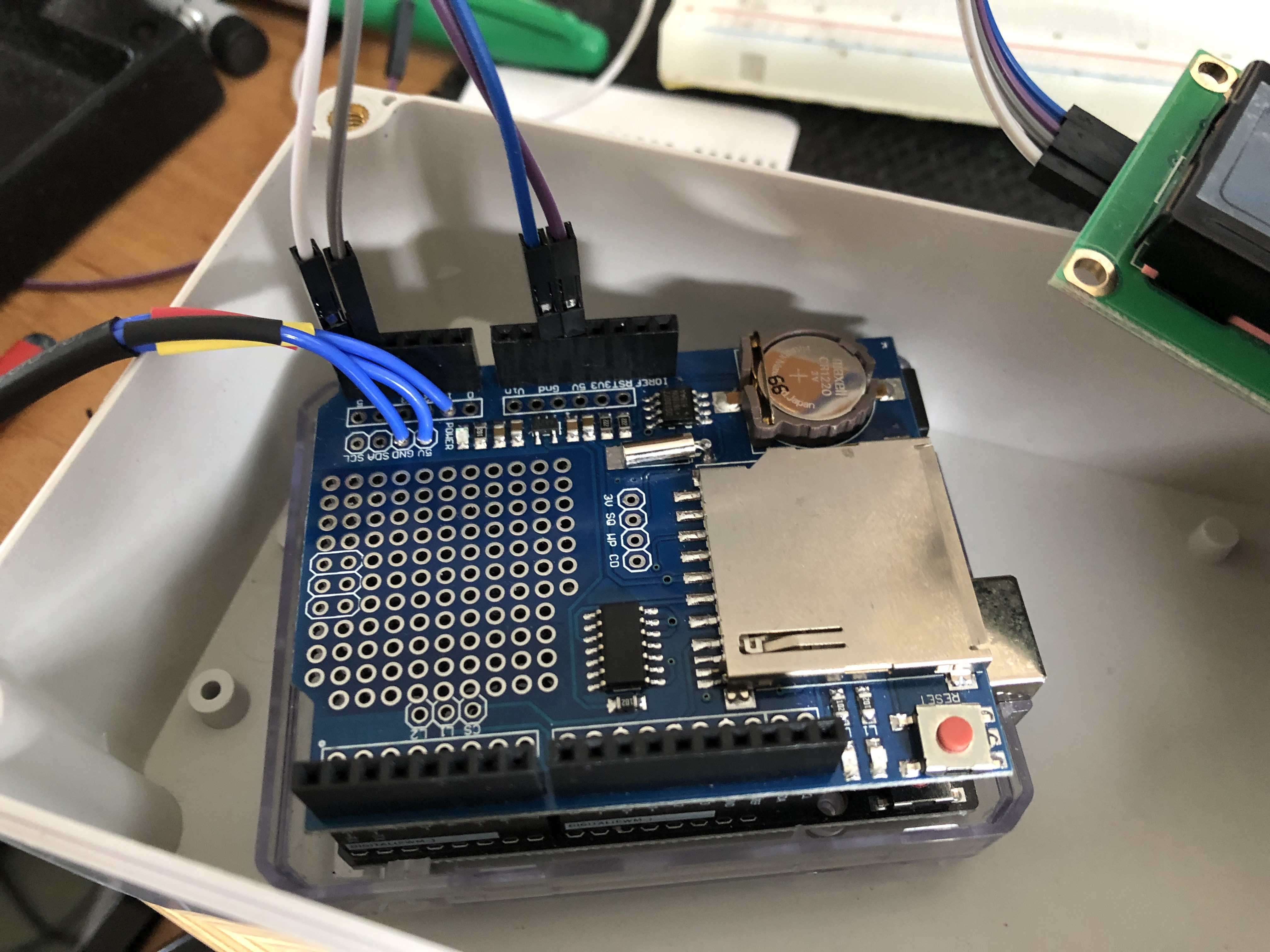A5 to SCL
A4 to SDA
5 volts to VCC
GND to GND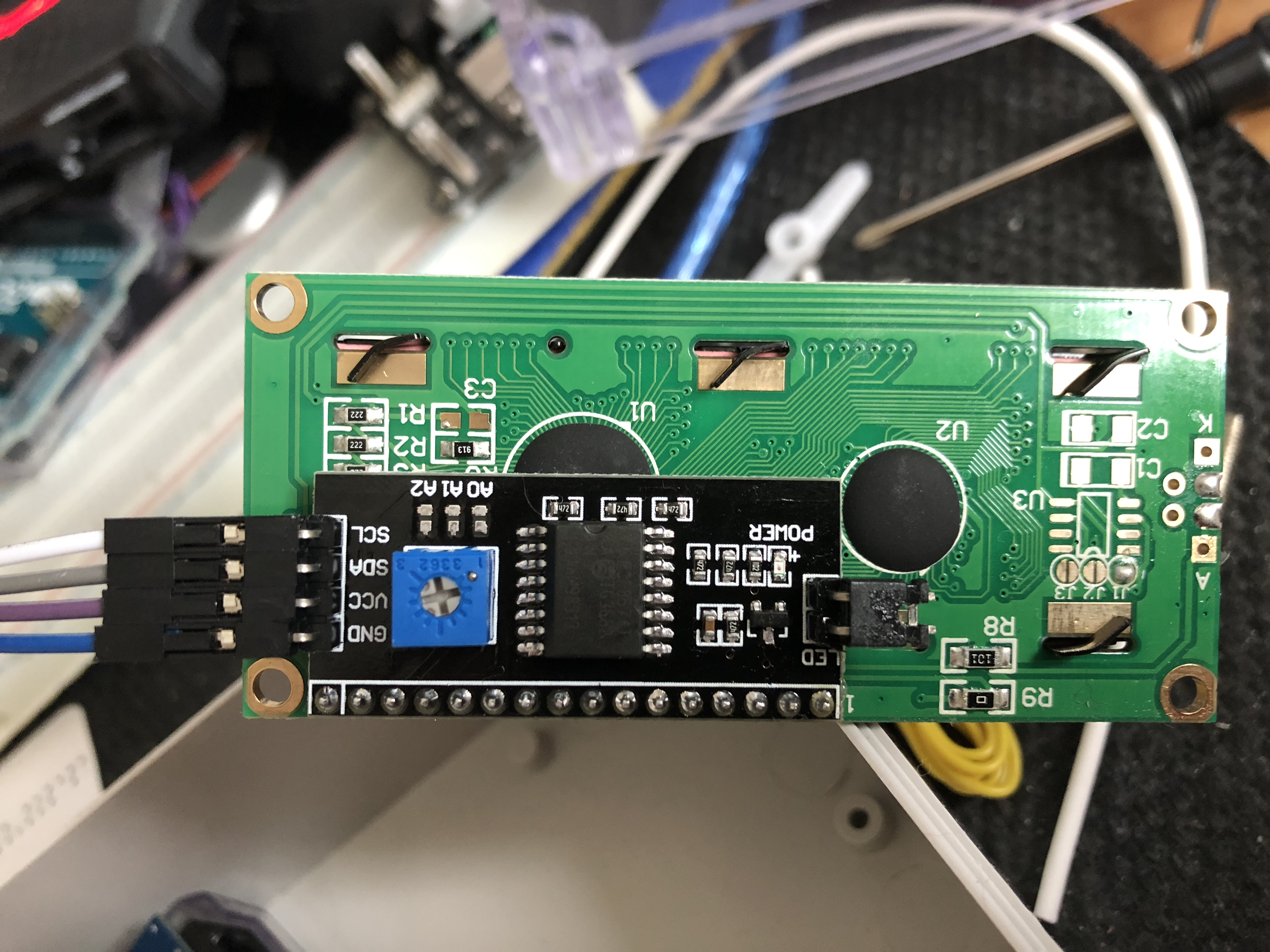yellow wire to A1
black wire to GND
red wire to 5 volts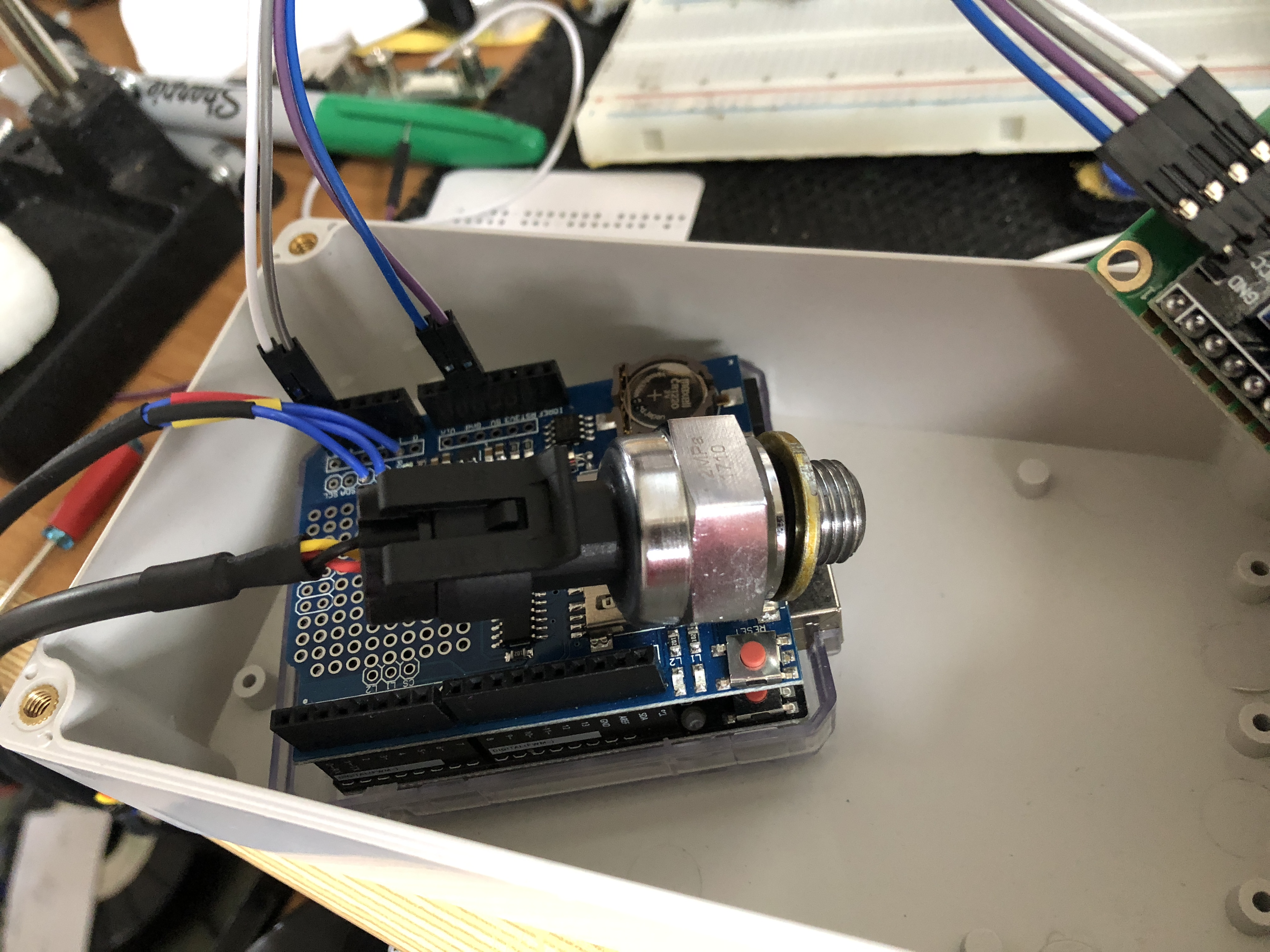connect to pc and confirm correct performance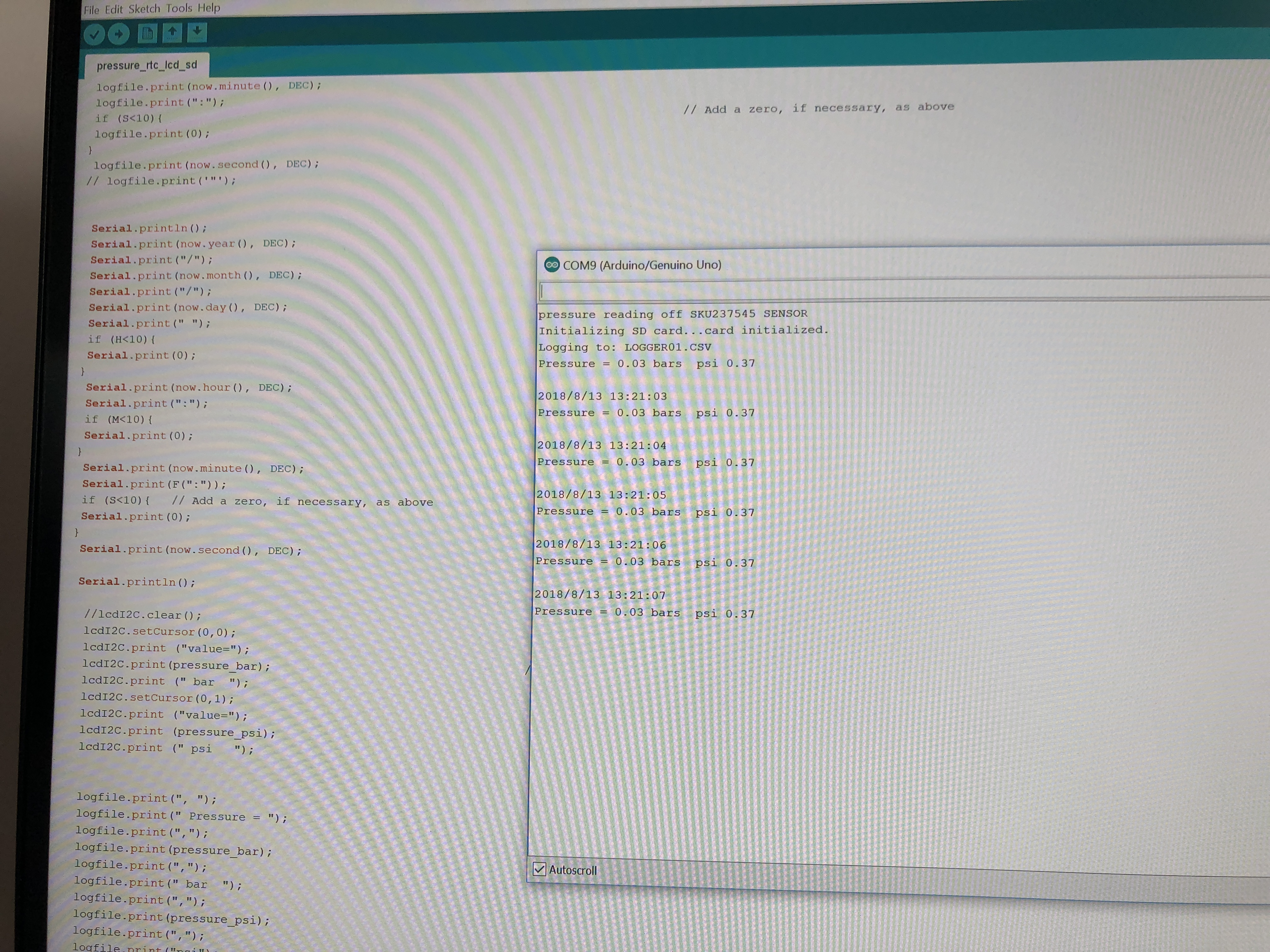#### BMP280: Measure Temperature, Pressure and Altitude

Project tutorial by SurtrTech

• 22,422 views
• 46 respects

#### Create a Diving Computer with an MS5803–01BA Pressure Sensor

Project showcase by Varul Jain

• 2,241 views
• 3 respects

#### IoT Pressure Sensor: MKR GSM + Arduino Cloud + Google Sheets

Project tutorial by wahltharvey

• 6,362 views
• 30 respects

#### Arduino-Based Pressure Cooker Whistle Indicator

Project tutorial by sourav344

• 2,135 views
• 8 respects

#### Measuring CO2 Levels aka "The Sleepiness Multiplier"

Project tutorial by Brian Carbonette

• 7,176 views